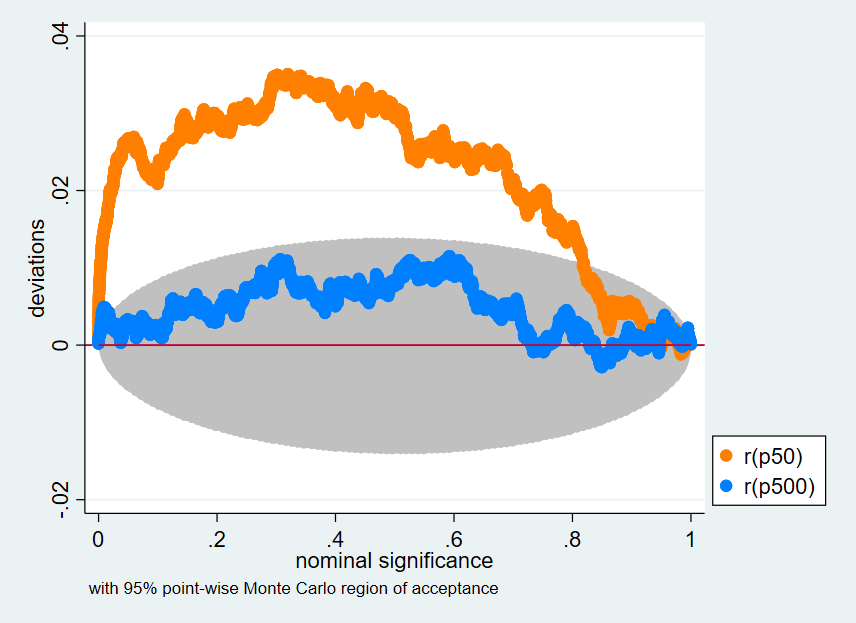Search

# Plot a Set of p-values From a Monte Carlo Simulation to Compare with the Nominal Significance

Stata has a command called simulate which is used to facilitate Monte Carlo simulations in Stata. Monte Carlo simulations are a method of analysis for solving computational or mathematical problems. You could use a Monte Carlo simulation to evaluate a theoretical probability. For example, we know theoretically that when you toss a coin there is a 50% chance you will get heads and a 50% chance you will get tails. To test whether this is observed in the real world you want to toss a coin 5000 times and record the outcome of each toss. However, it is very time consuming to do this. A Monte Carlo simulation offers a way to perform hundreds of thousands of individual coin tosses very quickly. For more information check out this Tech Tip where we use the Monte Carlo Method to try and determine the true value of Pi.

Once you have used simulate to generate a large number of simulated p-values, you can plot these visually use the simpplot command in Stata. This allows you to compare your actual values against the nominal significance values.

To create this plot in Stata, you first need to download the simpplot command from the SSC, which you do in Stata using the following command:To generate this graph in Stata, use the following commands: